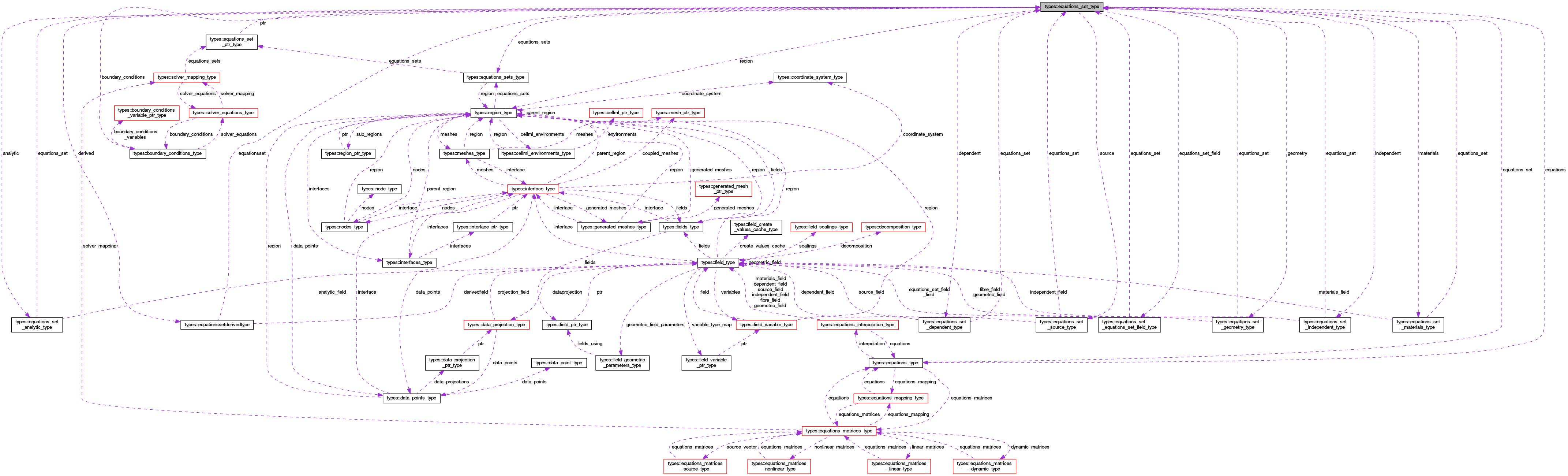OpenCMISS-Iron Internal API Documentation
types::equations_set_type Type Reference

Contains information on an equations set. More...

Collaboration diagram for types::equations_set_type:[legend]

## Public Attributes

integer(intg) user_number
The user identifying number of the equations set. More...

integer(intg) global_number
The global index of the equations set in the region. More...

logical equations_set_finished
Is .TRUE. if the equations set have finished being created, .FALSE. if not. More...

type(equations_sets_type), pointer equations_sets
A pointer back to the equations sets. More...

type(region_type), pointer region
A pointer back to the region containing the equations set. More...

integer(intg), dimension(:), allocatable specification
The equations set specification array, eg. [class, type, subtype], although there can be more or fewer identifiers. Unused identifiers are set to zero. More...

integer(intg) solution_method
The solution method for the equations set. More...

type(equations_set_geometry_typegeometry
The geometry information for the equations set. More...

type(equations_set_materials_type), pointer materials
A pointer to the materials information for the equations set. More...

type(equations_set_source_type), pointer source
A pointer to the source information for the equations set. More...

type(equations_set_dependent_typedependent
The depedent variable information for the equations set. More...

type(equations_set_independent_type), pointer independent
A pointer to the indepedent field information for the equations set. More...

type(equations_set_analytic_type), pointer analytic
A pointer to the analytic setup information for the equations set. More...

type(equationssetderivedtype), pointer derived
A pointer to the derived field information. More...

type(equations_type), pointer equations
A pointer to the equations information for the equations set. More...

type(boundary_conditions_type), pointer boundary_conditions
A pointer to the boundary condition information for the equations set. More...

type(equations_set_equations_set_field_typeequations_set_field
A pointer to the equations set field for the equations set. More...

## Detailed Description

Contains information on an equations set.

OPENCMISS::CMISSEquationsSetType

Definition at line 1941 of file types.f90.

## Member Data Documentation

 type(equations_set_analytic_type), pointer types::equations_set_type::analytic

A pointer to the analytic setup information for the equations set.

Definition at line 1954 of file types.f90.

 type(boundary_conditions_type), pointer types::equations_set_type::boundary_conditions

A pointer to the boundary condition information for the equations set.

Definition at line 1957 of file types.f90.

 type(equations_set_dependent_type) types::equations_set_type::dependent

The depedent variable information for the equations set.

Definition at line 1952 of file types.f90.

 type(equationssetderivedtype), pointer types::equations_set_type::derived

A pointer to the derived field information.

Definition at line 1955 of file types.f90.

 type(equations_type), pointer types::equations_set_type::equations

A pointer to the equations information for the equations set.

Definition at line 1956 of file types.f90.

 type(equations_set_equations_set_field_type) types::equations_set_type::equations_set_field

A pointer to the equations set field for the equations set.

Definition at line 1958 of file types.f90.

 logical types::equations_set_type::equations_set_finished

Is .TRUE. if the equations set have finished being created, .FALSE. if not.

Definition at line 1944 of file types.f90.

 type(equations_sets_type), pointer types::equations_set_type::equations_sets

A pointer back to the equations sets.

Definition at line 1945 of file types.f90.

 type(equations_set_geometry_type) types::equations_set_type::geometry

The geometry information for the equations set.

Definition at line 1949 of file types.f90.

 integer(intg) types::equations_set_type::global_number

The global index of the equations set in the region.

Definition at line 1943 of file types.f90.

 type(equations_set_independent_type), pointer types::equations_set_type::independent

A pointer to the indepedent field information for the equations set.

Definition at line 1953 of file types.f90.

 type(equations_set_materials_type), pointer types::equations_set_type::materials

A pointer to the materials information for the equations set.

Definition at line 1950 of file types.f90.

 type(region_type), pointer types::equations_set_type::region

A pointer back to the region containing the equations set.

Definition at line 1946 of file types.f90.

 integer(intg) types::equations_set_type::solution_method

The solution method for the equations set.

EQUATIONS_ROUTINES_SolutionMethods

Definition at line 1948 of file types.f90.

 type(equations_set_source_type), pointer types::equations_set_type::source

A pointer to the source information for the equations set.

Definition at line 1951 of file types.f90.

 integer(intg), dimension(:), allocatable types::equations_set_type::specification

The equations set specification array, eg. [class, type, subtype], although there can be more or fewer identifiers. Unused identifiers are set to zero.

Definition at line 1947 of file types.f90.

 integer(intg) types::equations_set_type::user_number

The user identifying number of the equations set.

Definition at line 1942 of file types.f90.